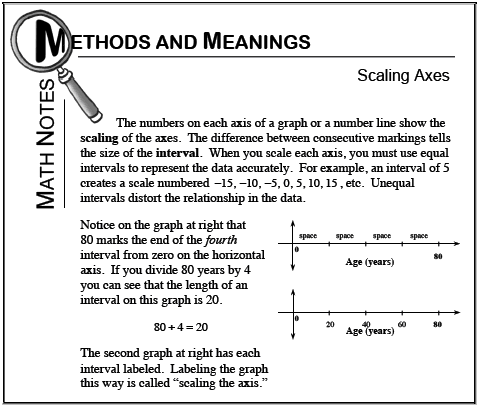### Home > CC2 > Chapter 1 > Lesson 1.2.2 > Problem1-74

1-74.

Read this lesson’s Math Notes box about scaling axes. Then, on your paper, copy the incomplete axes below and write the missing numbers on each one. Homework Help ✎

1.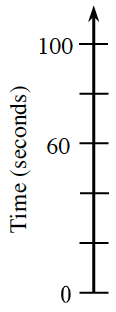See the Math Notes box below for this lesson.

Look at the two ticks labeled $60$ and $100$. What is the difference of $60$ and $100$? How many spaces are between them?

See the number line below.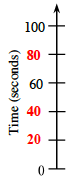1.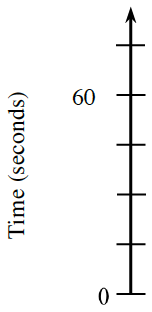See part (a).

1.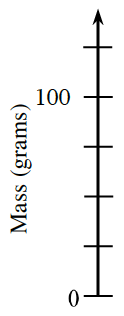See the number line below.

1.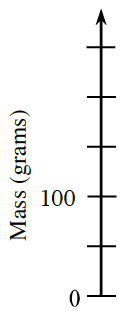See part (a).